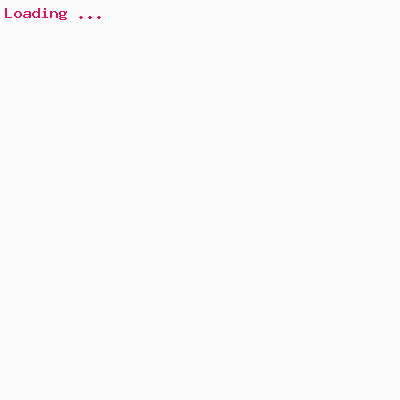## Bubble sort

Bubble sort compares the value of first element with the immediate next element and swaps according to the requirement and goes till the last element. This iteration repeates for (N - 1) times/steps where N is the number of elements in the list.Compare and swapping two elements like small soap bubbles and hence the name given as bubble sort.

## Bubble sort design

We are taking one inner loop for doing the exchange of the bubble. This loop will start from index 0 and take two immediate elements and swap if required. So this loop will iterate from 0 to a max of (count – 1) (note there are two elements).

### Inner bubble iteration & swap

for(index = 0; index < (count - step - 1); index++)
{

if (elements[index] > elements[index + 1])
{

swap_node(&elements[index],
&elements[index + 1]);
}

}

### Outer bubble sort steps

Now, this loop is needed for each step of the bubble sort. A bubble sort will require to iterate n-1 steps as the outer loop. For each step, it will go around to the inner bubble iteration & exchange steps.

for (step = 0; step < count - 1; step++)
{
/*Inner bubble iteration & swap */

}

### Bubble sort swap function

Bubble elements or two immediate elements can be exchanged via a swap function. This function should be a call be reference. We pass the address of two elements and this function will have a local variable to swap these two.

void swap_node(int *x, int *y)
{
int tmp;
tmp = *x;
*= *y;
*= tmp;
}

## Bubble sort example code

Here is a C source code of Bubble sorting with 5 integer elements. This code sorts the elements in ascending order.

/* Bubble sort example source code using C */
#include <stdio.h>

/* Swaps two elements */
void swap_node(int *x, int *y)
{
int tmp;
tmp = *x;
*= *y;
*= tmp;
}

/* Bubble sort example routine */
void bubble_sort(int elements[], int count)
{
int step, index;
printf("Bubble sort started-\n");
for (step = 0; step < count - 1; step++)
{
printf("==== Step %d ====\n", step+1);
print_array(elements, 5);
for(index = 0; index < (count - step - 1); index++)
{
printf("#%d ", index+1);
if (elements[index] > elements[index + 1])
{
printf(" swap %d > %d\n",
elements[index],
elements[index + 1]);
swap_node(&elements[index],
&elements[index + 1]);
} else
{
printf("no swap %d <= %d\n",
elements[index],
elements[index + 1]);
}
print_array(elements, 5);
}
}
printf("Bubble sort ended\n");

}

/* Prints the elements (for display purpose) */
void print_array(int array[], int count)
{
int i;
printf("[");
for(= 0; i < count; i++)
printf("%d, ", array[i]);
printf("\b\b]\n");
}

/* Main function */
int main(int argc, char *argv[])
{
int array = { 4, 9, 5, 1, 0 };
printf("Unsorted elements\n");
print_array(array, 5);
bubble_sort(array, 5);
printf("Bubble sorted elements\n");
print_array(array, 5);
}

## Steps and Iterations (diagrams)## Output

```Unsorted elements
[4, 9, 5, 1, 0]
Bubble sort started-
==== Step 1 ====
[4, 9, 5, 1, 0]
#1 no swap  4 <= 9
[4, 9, 5, 1, 0]
#2    swap  9 >  5
[4, 5, 9, 1, 0]
#3    swap  9 >  1
[4, 5, 1, 9, 0]
#4    swap  9 >  0
[4, 5, 1, 0, 9]
==== Step 2 ====
[4, 5, 1, 0, 9]
#1 no swap  4 <= 5
[4, 5, 1, 0, 9]
#2    swap  5 >  1
[4, 1, 5, 0, 9]
#3    swap  5 >  0
[4, 1, 0, 5, 9]
==== Step 3 ====
[4, 1, 0, 5, 9]
#1    swap  4 >  1
[1, 4, 0, 5, 9]
#2    swap  4 >  0
[1, 0, 4, 5, 9]
==== Step 4 ====
[1, 0, 4, 5, 9]
#1    swap  1 >  0
[0, 1, 4, 5, 9]
Bubble sort ended
Bubble sorted elements
[0, 1, 4, 5, 9]
```

## Efficiency and Big(O)

The efficiency of a sorting algorithm decides the performance of the algorithm when the total count of the elements reaches a large number. Bubble sort of N elements can take (N - 1) steps and (N -1) iterations in each step. Thus the total number of iterations needed for bubble sort is (N - 1)*(N - 1). This sorting algorithm is however not the best in performance when considering the scalability of the elements. The time complexity of bubble sort is O(N^2) [Square of N]. This sorting is well suited for a small number of elements and it is easy to implement in C or any other programming languages.